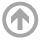Mathematics Department

# Expected Mathematical Background

These are topics Incoming USNA midshipmen are expected to be familiar with before arriving at the USNA. The precalculus exams you are required to take before arriving at USNA test these topics.

## Find the Equation of a Line

• using the point-intercept form, y = mx+b
• using the point-slope form (y-y0)/(x-x0) = m
• using the point-point form (y-y0)/(x-x0) = (y1-y0)/(x1-x0)
• parallel to a given line
• perpendicular to a given line.

## Basic Trigonometry

• the definitions of sin, cos, tan, cot, sec, csc
• Basic right-triangle trigonometry for sin-cos-tan in degrees and in radians,
• Trig values for 30-45-60-90 degree angles.
• The trig identity sin2(x) + cos2(x) = 1.

## Basic Arithmetic with Fractions (of both numbers and polynomials)

• Adding, Subtracting, Multiplying, Dividing, Finding common denominator of two fractions.
• Rationalize numerator and denominator containing a square root.

## Polynomial Arithmetic

• completing the square
• long division of polynomials
• expanding a product of linear functions using FOIL (first, outside, inside, last).
• the meaning of a "solution of a polynomial equation".

## Basics of Functions

• the concept of a real-valued function - range and domain.
• Know the graphs of ""basic" functions, such as:
• Trig functions (cos, sin, tan)
• natural log ln and the exponential ex
• x2, x3, 1/x, x1/2
• Laws for exponentials (ca+b=ca cb, etc).
• Laws for logarithms (ln (ab) = ln(a)+ln(b), etc).

## Resources

If you don't know these topics well enough, here are some references to help you review before taking the online exams.